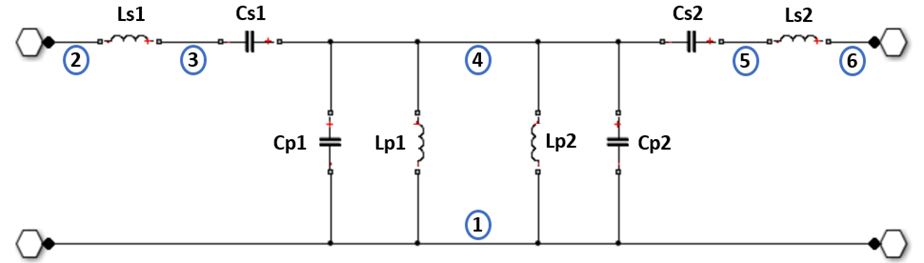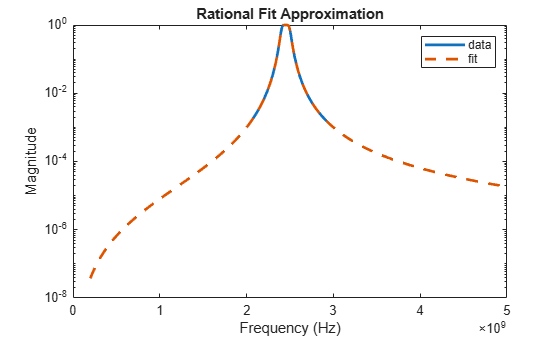# Bandpass Filter Response

This example shows how to compute the time-domain response of a simple bandpass filter. The eight steps involved in computing the time-domain response of a simple bandpass filter are,

1. Use the classic image parameter design to assign inductance and capacitance values to the bandpass filter.

2. Use the `circuit`, `capacitor`, and `inductor` objects with the `add` function to programmatically construct a Butterworth circuit.

3. Use the `setports` function to define the circuit as a 2-port network.

4. Use the `sparameters` function to extract the S-parameters of the 2-port network over a wide frequency range.

5. Use the `s2tf` function to compute the voltage transfer function from the input to the output.

6. Use the `rational` object to generate rational fits that capture the ideal RC circuit to a very high degree of accuracy.

7. Use the `randn` function to create noise in order to create a noisy input voltage waveform.

8. Use the `timeresp`` function` to compute the transient response to a noisy input voltage waveform.

### Design Bandpass Filter Using Image Parameters

The image parameter design is a framework for analytically computing the values of the series and parallel components in the passive filters. For more information on Image Parameters, see "Complete Wireless Design" by Cotter W. Sayre, McGraw-Hill 2008 p. 331.Figure 1: A Butterworth bandpass filter built out of two half-sections.

Generate the component values for a bandpass filter with a lower 3 dB cutoff frequency of 2.4 GHz and an upper 3 dB cutoff frequency of 2.5 GHz.

```Ro = 50; f1C = 2400e6; f2C = 2500e6; Ls = (Ro / (pi*(f2C - f1C)))/2; % Ls1 and Ls2 Cs = 2*(f2C - f1C)/(4*pi*Ro*f2C*f1C); % Cs1 and Cs2 Lp = 2*Ro*(f2C - f1C)/(4*pi*f2C*f1C); % Lp1 and Lp2 Cp = (1/(pi*Ro*(f2C - f1C)))/2; % Cp1 and Cp2```

### Programmatically Construct Circuit

Before building the circuit using the `inductor` and `capacitor` objects, nodes in the circuit are numbered. This is shown in figure 1.Figure 2: Node numbers added to the Butterworth bandpass filter.

Create a `circuit` object and populate it with the `inductor` and the `capacitor` objects using the `add` function.

```ckt = circuit('butterworthBPF'); add(ckt,[3 2],inductor(Ls)); % Ls1 add(ckt,[4 3],capacitor(Cs)); % Cs1 add(ckt,[5 4],capacitor(Cs)); % Cs2 add(ckt,[6 5],inductor(Ls)); % Ls2 add(ckt,[4 1],capacitor(Cp)); % Cp1 add(ckt,[4 1],inductor(Lp)); % Lp1 add(ckt,[4 1],inductor(Lp)); % Lp2 add(ckt,[4 1],capacitor(Cp)); % Cp2```

### Extract S-Parameters From 2-Port Network

To extract S-parameters from the circuit object, first use the `setports` function to define the circuit as a 2-port network.

`freq = linspace(2e9,3e9,101);`

Use the `sparameters` function to extract the S-parameters at the frequencies of interest.

```setports(ckt,[2 1],[6 1]) S = sparameters(ckt,freq);```

### Fit Transfer Function of Circuit to Rational Function

Use the `s2tf` function to generate a transfer function from the S-parameter object.

`tfS = s2tf(S);`

Use the `rational` object to fit the transfer function data to a rational function.

`fit = rational(freq,tfS);`

### Verify Rational Fit Approximation

Use the `freqresp` function to verify that the rational fit approximation has reasonable behavior outside both sides of the fitted frequency range.

```widerFreqs = linspace(2e8,5e9,1001); resp = freqresp(fit,widerFreqs);```

Plot to visualize rational fit approximation. The rational fit behaves well outside the fitted frequency range.

```figure semilogy(freq,abs(tfS),widerFreqs,abs(resp),'--','LineWidth',2) xlabel('Frequency (Hz)'); ylabel('Magnitude'); legend('data','fit'); title('Rational Fit Approximation');```### Construct Input Signal to Test Bandpass Filter

To test the bandpass filter, designed by the Image Parameter technique, a sinusoidal signal at 2.45 GHz is recovered from the noisy input signal. The noise input signal is generated by the inclusion of zero-mean random noise and a blocker at 2.35 GHz to the input signal.

Construct a input and a noisy input signal with 8192 samples.

```fCenter = 2.45e9; fBlocker = 2.35e9; period = 1/fCenter; sampleTime = period/16; signalLen = 8192; t = (0:signalLen-1)'*sampleTime; % 256 periods input = sin(2*pi*fCenter*t); % Clean input signal rng('default') noise = randn(size(t)) + sin(2*pi*fBlocker*t); noisyInput = input + noise; % Noisy input signal```

### Compute Transient Response to Input Signal

Use the `timeresp` function to compute the analytic solutions of the state-space.

`output = timeresp(fit,noisyInput,sampleTime);`

### View Input Signal and Filter Response in Time Domain

Plot the input signal, noisy input signal, and the band pass filter output in a figure window.

```xmax = t(end)/8; figure subplot(3,1,1) plot(t,input) axis([0 xmax -1.5 1.5]) title('Input') subplot(3,1,2) plot(t,noisyInput) axis([0 xmax floor(min(noisyInput)) ceil(max(noisyInput))]); title('Noisy Input'); ylabel('Amplitude (volts)'); subplot(3,1,3) plot(t,output) axis([0 xmax -1.5 1.5]); title('Filter Output'); xlabel('Time (sec)');```### View Input Signal and Filter Response in Frequency Domain

Overlaying the noisy input and the filter response in the frequency domain explains why the filtering operation is successful. Both the blocker signal at 2.35 GHz and much of the noise are significantly attenuated.

```NFFT = 2^nextpow2(signalLen); % Next power of 2 from length of y Y = fft(noisyInput,NFFT)/signalLen; samplingFreq = 1/sampleTime; f = samplingFreq/2*linspace(0,1,NFFT/2+1)'; O = fft(output,NFFT)/signalLen; figure subplot(2,1,1) plot(freq,abs(tfS),'b','LineWidth',2) axis([freq(1) freq(end) 0 1.1]); legend('filter transfer function'); title('Transfer function of Bandpass filter'); ylabel('Magnitude'); subplot(2,1,2) plot(f,2*abs(Y(1:NFFT/2+1)),'g',f,2*abs(O(1:NFFT/2+1)),'r','LineWidth',2) axis([freq(1) freq(end) 0 1.1]); legend('input+noise','output'); title('Filter characteristic and noisy input spectrum.'); xlabel('Frequency (Hz)'); ylabel('Magnitude (Volts)');```To compute and display this bandpass filter response using RFCKT objects, see Bandpass Filter Response using RFCKT Objects.